GROUP THEORY SYMBOL

# GTPartialDOS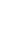GTPartialDOS[Hamiltonian,structure,parameters]
calculates and plots the partial density of states for a given Hamiltonian.

## DetailsDetails

• The density of states (DOS) is calculated by a simple Gaussian broadening method.
• structure defines the Bravais lattice. This information is used to construct the k-mesh in the irreducible part of the Brillouin zone.
• The vector parameters contains data for the DOS calculation.
• Note, this works only for tight-binding Hamitonians with a well defined orbital characters.
• parameters = {nt, scaleK, emin, emax, ne, σ, scale}
•  nt refinement parameter k-mesh (see also GTBZPointMesh) scaleK scaling factor k-mesh (see also GTBZPointMesh) [emin,emax] energy intervall for DOS calculation ne number of energy points to calculate the DOS σ width of the Gaussians (if σ = 0 the energy step width is used) scale The whole DOS can be scaled by the factor scale. This is sometimes helpful for comperisons. {{"p",{2,3,4}} defines the orbitals to be used in the pDOS plot
• The following options can be given:
•  FrameLabel {"Energy", "DOS"} Option of ListPlot GOFermiEnergy -100000 Calculates the Fermi energy GOPlotDos "DOS" Plots density of states GOStore 0 Stores the DOS, if a file name is given PlotLabel "Density of states" Option of ListPlot PlotRange All Option of ListPlot PlotStyle RGBColor[0, 0, 1] Option of ListPlot
• See: W. Hergert, M. Geilhufe, Group Theory in Solid State Physics and Photonics. Problem Solving with Mathematica
• This is a new command introduced in version 1.0.1. A detailed description does not appear in the book.

## ExamplesExamplesopen allclose all

### Basic Examples  (1)Basic Examples  (1)

 In:=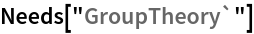In:=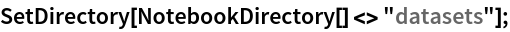As an example, the DOS of Cu is calculated. We import the Hamiltonian and the corresponding parameters from a dataset.

 In:=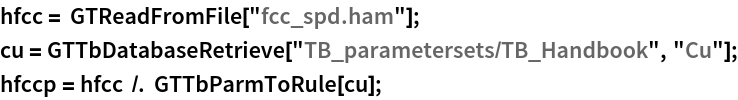For the partial DOS calculation, we provide a parameter list, where the last item describes which partial densities of states are calculated.

 In:=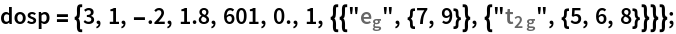The partial density of states in k-space is calculated using GTPartialDOS.

 In:=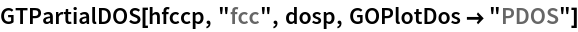Out=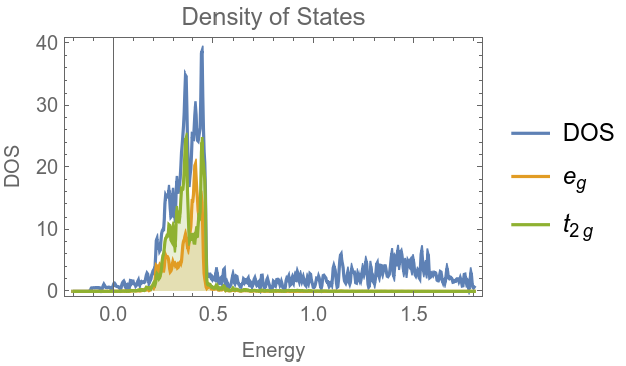### Options  (4)Options  (4)

GTBandsGTBandStructureGTDensityOfStatesPlotGTTbHamiltonianGTBZPointMeshGTFatBandsPlotGTDensityOfStatesGTBandsDOSPlot